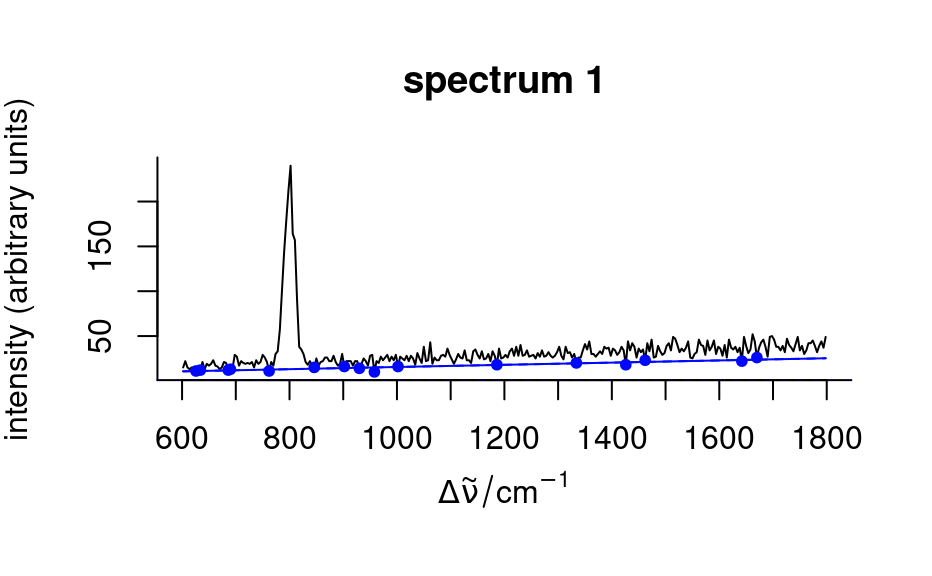These functions fit polynomial baselines.

spc_fit_poly(
fit.to,
apply.to = NULL,
poly.order = 1,
offset.wl = !(is.null(apply.to))
)

spc_fit_poly_below(
fit.to,
apply.to = fit.to,
poly.order = 1,
npts.min = max(round(nwl(fit.to) * 0.05), 3 * (poly.order + 1)),
noise = 0,
offset.wl = FALSE,
max.iter = nwl(fit.to),
stop.on.increase = FALSE,
debuglevel = hy_get_option("debuglevel")
)

Arguments

fit.to

hyperSpec object on which the baselines are fitted

apply.to

hyperSpec object on which the baselines are evaluted If NULL, a hyperSpec object containing the polynomial coefficients rather than evaluted baselines is returned.

poly.order

order of the polynomial to be used

offset.wl

should the wavelength range be mapped to -> [0, delta wl]? This enhances numerical stability.

npts.min

minimal number of points used for fitting the polynomial

noise

noise level to be considered during the fit. It may be given as one value for all the spectra, or for each spectrum separately.

max.iter

stop at the latest after so many iterations.

stop.on.increase

additional stopping rule: stop if the number of support points would increase, regardless whether npts.min was reached or not.

debuglevel

additional output: 1 shows npts.min, 2 plots support points for the final baseline of 1st spectrum, 3 plots support points for 1st spectrum, 4 plots support points for all spectra.

Value

hyperSpec object containing the baselines in the spectra matrix, either as polynomial coefficients or as polynomials evaluted on the spectral range of apply.to

Details

Both functions fit polynomials to be used as baselines. If apply.to is NULL, a hyperSpec object with the polynomial coefficients is returned, otherwise the polynomials are evaluated on the spectral range of apply.to.

spc_fit_poly() calculates the least squares fit of order poly.order to the complete spectra given in fit.to. Thus fit.to needs to be cut appropriately.

spc_fit_poly_below() tries to fit the baseline on appropriate spectral ranges of the spectra in fit.to. For details, see the vignette("baseline").

C. Beleites

Examples

if (FALSE) {
vignette("baseline", package = "hyperSpec")
}

spc <- faux_cell[1:10]
baselines <- spc_fit_poly(spc[, , c(625 ~ 640, 1785 ~ 1800)], spc)
plot(spc - baselines)baselines <- spc_fit_poly_below(spc)
plot(spc - baselines)spc_fit_poly_below(faux_cell[1:3], debuglevel = 1)
#> Fitting with npts.min = 15
#> spectrum      1:    29 support points, noise = 0.0,   4 iterations
#> spectrum      2:    19 support points, noise = 0.0,   5 iterations
#> spectrum      3:    31 support points, noise = 0.0,   4 iterations
#> hyperSpec object
#>    3 spectra
#>    4 data columns
#>    300 data points / spectrum
spc_fit_poly_below(faux_cell[1:3], debuglevel = 2)
#> Fitting with npts.min = 15
#> start: 300 support points
#> spectrum      1:    29 support points, noise = 0.0,   4 iterations
#> spectrum      2:    19 support points, noise = 0.0,   5 iterations
#> spectrum      3:    31 support points, noise = 0.0,   4 iterations#> hyperSpec object
#>    3 spectra
#>    4 data columns
#>    300 data points / spectrum
spc_fit_poly_below(faux_cell[1:3],
debuglevel = 3,
noise = sqrt(rowMeans(faux_cell[[1:3]]))
)
#> Fitting with npts.min = 15
#> start: 300 support points
#> Iteration 1: 259 support points
#> Iteration 2: 230 support points
#> Iteration 3: 212 support points
#> Iteration 4: 209 support points
#> Iteration 5: 206 support points
#> Iteration 6: 206 support points
#> spectrum      1:   206 support points, noise = 14.6,   6 iterations
#> spectrum      2:   205 support points, noise = 6.5,   6 iterations
#> spectrum      3:   208 support points, noise = 13.8,   6 iterations#> hyperSpec object
#>    3 spectra
#>    4 data columns
#>    300 data points / spectrum